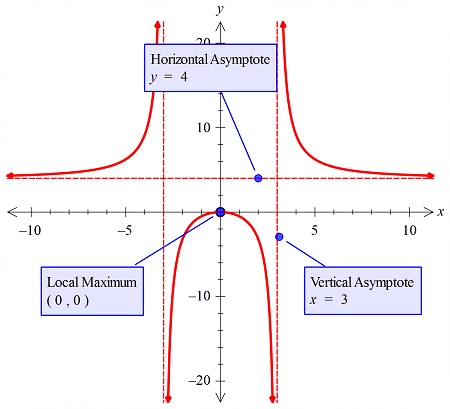# Sketch the graph of f(x) = frac{4x^2}{x^2 - 9} showing any asymptotes, intercepts,...

## Question:

Sketch the graph of {eq}f(x) = \frac{4x^2}{x^2 - 9} {/eq} showing any asymptotes, intercepts, increasing/decreasing, extrema, concavity, and points of inflection.

## Graphs:

The graph of the function that has the maxima or the minima and the asymptotes, may or may not have the point of inflection. But the concavity may change because of the presence of discontinuity points.

## Answer and Explanation:

In the problem, we have to plot the graph and then show the points, so we plotted the graph below:#### Learn more about this topic:Graphs: Types, Examples & Functions

from High School Algebra II: Help and Review

Chapter 16 / Lesson 11
355K